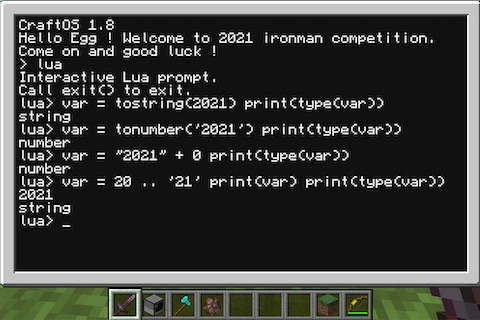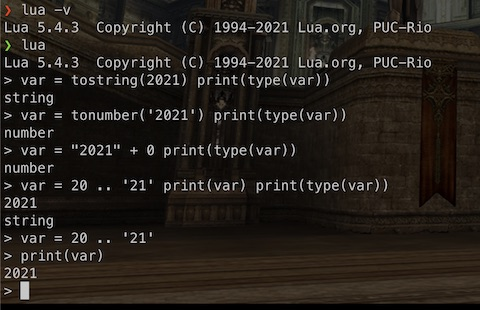#DAY 5
0
Software Development

## Day5 「開機」學習 Lua - 變數型別與宣告

motd.lua

``````local date = os.date("*t")
if date.month == 1 and date.day == 1 then
print("Happy new year!")
elseif date.month == 12 and date.day == 24 then
print("Merry X-mas!")
elseif date.month == 10 and date.day == 31 then
print("OOoooOOOoooo! Spooky!")
else
local tMotd = {}

for sPath in string.gmatch(settings.get("motd.path"), "[^:]+") do
if fs.exists(sPath) then
for sLine in io.lines(sPath) do
table.insert(tMotd, sLine)
end
end
end

if #tMotd == 0 then
print("missingno")
else
print(tMotd[math.random(1, #tMotd)])
end
end
``````

### 變數範圍

``````function showTitle()
title = 'ironman'
end

showTitle()
print(title)
``````

### 變數型別與宣告

``````var = nil
print(type(var))  -- nil，變數預設值，變數未經宣告直接取值也是 nil
var = true
print(type(var))  -- boolean
var = 30
print(type(var))  -- number
var = 'ironman'
print(type(var))  -- string，可以用單引號說雙引號
var = function (n) return n*2 end
print(type(var))  -- function
var = coroutine.create(function() print("Hello, ironman") end)
var = {}
print(type(var))  -- table
``````

### 型別轉換

``````var = tostring(2021)
print(type(var))
var = tonumber('2021')
print(type(var))
``````

``````var = 20 .. 21
print(var)
var = 2020 + '1'
print(var)
``````